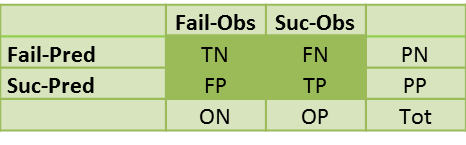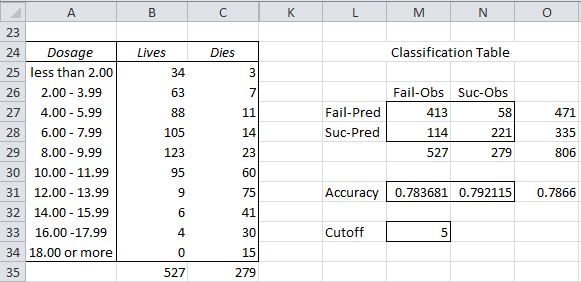# Classification Table

The Classification Table compares the predicted number of successes  to the number successes actually observed and similarly the predicted number of failures compared to the number actually observed.

We have four possible outcomes:

True Positives (TP) = the number of cases which were correctly classified to be positive, i.e. were predicted to be a success and were actually observed to be a success

False Positives (FP) = the number of cases which were incorrectly classified as positive, i.e. were predicted to be a success but were actually observed to be a failure

True Negatives (TN) = the number of cases which were correctly classified to be negative, i.e. were predicted to be a failure and were actually observed to be a failure

False Negatives (FN) = the number of cases which were incorrectly classified as negative, i.e. were predicted to be a negative but were actually observed to be a success

The Classification Table takes the formwhere PP = predicted positive = TP + FP, PN = predicted negative = FN + TN, OP = observed positive = TP + FN, ON = observed negative = FP + TN and Tot = the total sample size = TP + FP + FN + TN.

Example 1: A new spray is being tested for killing mosquitos. In particular the researchers want to discover the correct dosage of the spray. They tested 806 mosquitos with dosages varying from 0 μg to 20 μg and tabulated the number of mosquitos who died and lived in 2 μg dosage intervals as shown in range A24:C34 of Figure 1.

Create the classification table for a dosage of 10 μg or more. Success is viewed as mosquito died and failure is mosquito lived. A dosage of less than 10 μg is viewed as a prediction of failure (mosquito lives) and a dosage of 10 μg or more is viewed as a prediction of success (mosquito dies).Figure 1 – Classification Table

We will use a cutoff value which corresponds to the last row in the table that is a failure, which in this case is row 5 (8.00 – 9.99).

For the data in Figure 1 we have

TN = 413 (cell M27), which can be calculated by the formula =SUM(B25:B29)

FN = 58 (cell N27), which can be calculated by the formula =SUM(C25:C29)

FP = 114 (cell M28), which can be calculated by the formula =B35-M27

TP = 221 (cell N28), which can be calculated by the formula = C35-N27

Note that FP is the type I error and FN is the type II error as described in Hypothesis Testing.

We now can define the following:

True Positive Rate (TPR), aka Sensitivity = TP/OP = 221/279 = .792115 (cell N31)

True Negative Rate (TNR), aka Specificity = TN/ON = 413/527 = .783681 (cell M31)

Accuracy (ACC) = (TP + TN)/Tot = (221+413) / 806 = .7866 (cell O31)

False Positive Rate (FPR) = 1 – TNR = FP/ON = 114/527 = .216319

Positive Predictive Value (PPV) = TP/PP = 221/335 = .659701

Negative Predictive Value (NPV) = TN/PN = 413/471 = .876858

Accuracy is a measure of the fit of the model (i.e. a dosage of 10 μg or more in this example). For Example 1 this is .7866, which means that the model gives an accurate prediction 78.66% of the time, or simply stated 78.66% of the mosquitos show the right outcome: they die when the dosage is 10 μg or more and live when the dosage is less than 10 μg.

# Personal Career & Learning Guide for Data Analyst, Data Engineer and Data Scientist

## Applied Machine Learning & Data Science Projects and Coding Recipes for Beginners

A list of FREE programming examples together with eTutorials & eBooks @ SETScholars

# Projects and Coding Recipes, eTutorials and eBooks: The best All-in-One resources for Data Analyst, Data Scientist, Machine Learning Engineer and Software Developer

Topics included: Classification, Clustering, Regression, Forecasting, Algorithms, Data Structures, Data Analytics & Data Science, Deep Learning, Machine Learning, Programming Languages and Software Tools & Packages.
(Discount is valid for limited time only)`Disclaimer: The information and code presented within this recipe/tutorial is only for educational and coaching purposes for beginners and developers. Anyone can practice and apply the recipe/tutorial presented here, but the reader is taking full responsibility for his/her actions. The author (content curator) of this recipe (code / program) has made every effort to ensure the accuracy of the information was correct at time of publication. The author (content curator) does not assume and hereby disclaims any liability to any party for any loss, damage, or disruption caused by errors or omissions, whether such errors or omissions result from accident, negligence, or any other cause. The information presented here could also be found in public knowledge domains.`

# Learn by Coding: v-Tutorials on Applied Machine Learning and Data Science for Beginners

Please do not waste your valuable time by watching videos, rather use end-to-end (Python and R) recipes from Professional Data Scientists to practice coding, and land the most demandable jobs in the fields of Predictive analytics & AI (Machine Learning and Data Science).

The objective is to guide the developers & analysts to “Learn how to Code” for Applied AI using end-to-end coding solutions, and unlock the world of opportunities!